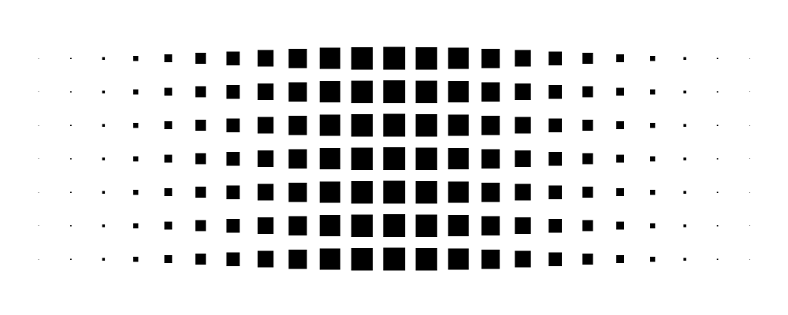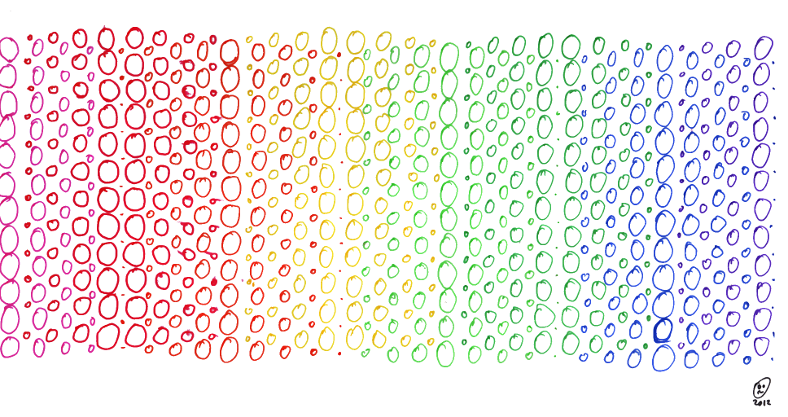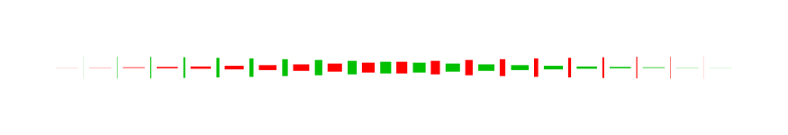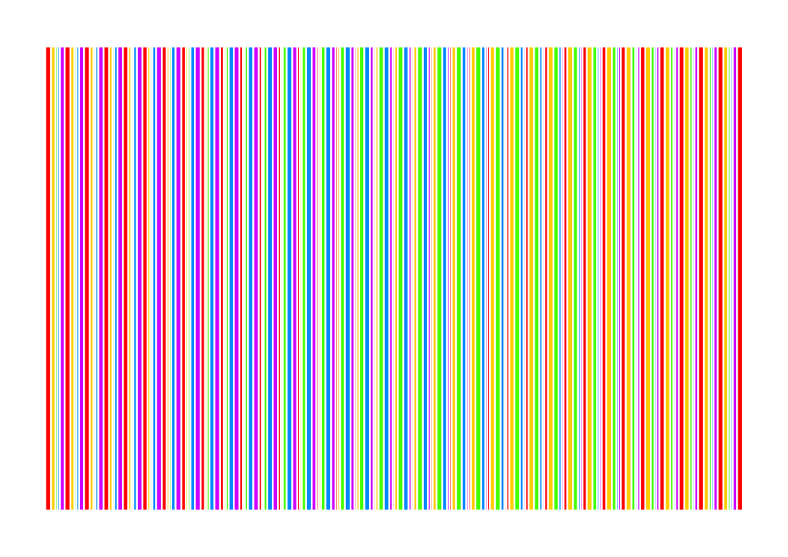# mathr / blog / #

## LozengeBased on a drawing from a year an a half ago:I showed it to Brent Yorgey in the #diagrams IRC channel and he made this:

strip :: (Double -> Double) -> Double -> Double -> Int -> Double -> Diagram SVG R2
strip f lo hi n offset
= [lo, lo + (hi - lo) / (fromIntegral n - 1) .. hi]
# map (square . f)
# hcat' with {sep = offset, catMethod = Distrib}
# fc black

example = vcat' with { sep = 3, catMethod = Distrib } (replicate 7 str)
where str = strip (\x -> cos x + 1) (-pi) pi 23 3
--Lozenge (Brent Yorgey)

Then I showed him how they interleave and he made this:

strip :: (Double -> Double) -> Double -> Double -> Int -> Double -> Diagram SVG R2
strip f lo hi n offset
= [lo, lo + (hi - lo) / (fromIntegral n - 1) .. hi]
# map (square . f)
# hcat' with {sep = offset, catMethod = Distrib}

lozenge = vcat' with { sep = 3, catMethod = Distrib } (replicate 7 str)
# centerXY
where str = strip (\x -> cos x + 1) (-pi) pi 23 3

example = mconcat
[ lozenge # fc black
, lozenge # fc red
# translateY (-1.5)
# translateX (width lozenge / 2 - 4.5)
]
--Lozenges (Brent Yorgey)

These are so much simpler than my original code which was horrible C numerical stuff to converge a waveform to the right shape, printing numbers which I fed into GNUplot and then editing the image with GIMP to correct the aspect ratio. I don't have that original code any more, but here's a similar version:

#include <math.h>
#include <stdio.h>
#include <stdlib.h>

const double pi = 3.141592653589793;

int cmp(const void *x, const void *y) {
const double *a = x;
const double *b = y;
if (*a < *b) return -1;
if (*a > *b) return 1;
return 0;
}

double y(double x) {
return (cos(pi * x) + 1) / 2;
}

int main(int argc, char **argv) {
if (argc < 2) {
return 1;
}
int n = atoi(argv);
double a;
double x[n+1];
int w = 0;
a = 0.001;
for (int i = 0; i <= n; ++i) {
x[i] = (2.0 * i) / (2.0 * n + 1.0);
}
x = 0;
while (1) {
printf("a\t= %g\n", a[w]);
for (int i = 0; i <= n; ++i) {
printf("x[%d]\t= %g\t%g\n", i, x[w][i], y(x[w][i]));
}
double s = y(x[w]);
for (int i = 1; i <= n; ++i) {
s += 2 * y(x[w][i]);
}
a[1 - w] = 1 / s;
for (int i = 1; i <= n; ++i) {
x[1 - w][i] = x[w][i-1] + a[w] *
((y(x[w][i-1]) + y(x[w][i]))/2 + y(x[w][n+1-i]));
}
w = 1 - w;
qsort(&x[w], n+1, sizeof(x[w]), cmp);
}
return 0;
}
gcc -std=c99 -lm lozenge.c
./a.out 19 | head -n 2100 | tail -n 20 > lozenge.dat ; gnuplot
unset key
unset xtics
unset ytics
unset border
set style fill solid
set terminal png size 8192,8192
set output "lozenge-raw.png"
plot [-0.2:1.2] [-0.7:0.7] "lozenge.dat" using 3:(0):\
($3 -$4 * 0.0512405/3):($3 +$4 * 0.0512405/3):\
((-1+$4) * 0.0512405/3):((1-$4)  * 0.0512405/3) with boxxyerrorbars,\
"lozenge.dat" using (1-$3):(0):\ (1-$3 - $4 * 0.0512405/3):(1-$3 + $4 * 0.0512405/3):\ ((-1+$4)   * 0.0512405/3):((1-\$4)    * 0.0512405/3) with boxxyerrorbars
gimp (crop image)A further experiment led to this gradient with 5 colours out of phase:Do check out the links above to the Haskell diagrams pastebin, it's quite awesome.